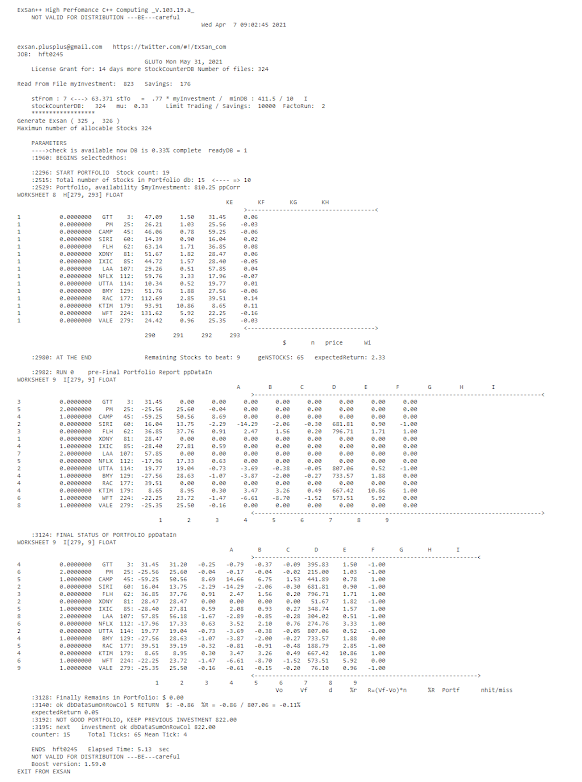## Posts

### Markowitz Portfolio Simulator### Well, It is not an integerExSan++ Exsan High Perfomance C++ Computing_V.7.25.a_ iTwit         exsan.plusplus@gmail.com   Fri Apr 10 15:24:44 2020         x: 18.10.25        c:\exsan    JOB ID: intSCP_B2444 REFERENCE: This is an Integer! UNBELIEVABLE!    ////***snippetCodeBegin unsigned short i(160), pW(5), sW(15) ; double x, y, z; string sq_x = "sqrt of x"; string pisq_x = "pi*(sqrt_x)"; for (; i <= 165; i++) {     x[i] = double(i);     y[i] = pi * sqrt(x[i]);     y[i] = pow(exp(1), y[i]);     cout << "\ny = e ^ (pi * sqrt(x)) = " << std::fixed << setw(50) << setprecision(10) << y[i];          z[i] = pow(log(y[i])/pi, 2.0);     if (z[i] == i) {         cout << "\nThis is a valid output";     }     else {         cout << "\nThis is a NOTvalid outputexpected X was " << i;     } } cpp_dec_f

### this is an integer indeed, is'n it?ExSan++ Exsan High Perfomance C++ Computing_V.7.25.a_ iTwit         exsan.plusplus@gmail.com   Wed Apr 8 10:41:05 2020         x: 18.10.25        c:\exsan    JOB ID: intSCP4105 — Tamás Görbe (@TamasGorbe) March 31, 2020 " ////***snippetCodeBegin // if val of variable is zero then false double y(0); // if val of variable is zero then false // if differente from zero then true if (y){      cout << "\ny = " << "TRUE y != 0"; } else {      cout << "\ny = " << "FALSE y = 0"; } //lets make y != 0 y = 0.000000000000001; if (y) {      cout << "\ny = " << "TRUE y != 0"; } else {      cout << "\ny = " << "FALSE y = 0"; } //test format y = 0.0000000000000000000000001; cout &